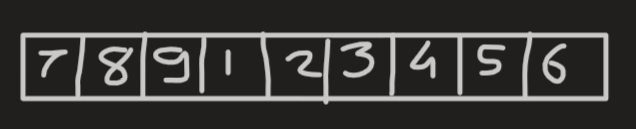# Left rotate an array by D places in C++

Hello, friends in this tutorial we will learn to left rotate an array by D places and write the code for it in C++.

An array is a collection of elements of similar data types stored in contiguous memory locations. It is used to store a collection of data. We can access the elements of an array by the index at which the element is stored.

Given an integer array of N elements, we have to rotate the array by D places.

For example, consider the following array of 9 elements from 1 to 9 and D=6Hence after rotating the array by 6 places we get the following resultant array.The approach is simple, consider Input array arr[] = [1, 2, 3, 4, 5, 6, 7, 8, 9], d = 6, n = 9

1. Store the first d elements in a temporary array temp[] = [1, 2, 3, 4, 5, 6]
2. Shift the remaining elements of the array toward the start of the array.
3. Store back the d elements to the array arr[] = [7, 8, 9, 1, 2, 3, 4, 5, 6]

## C++ program to rotate the array by D places

Following is the code to left rotate the array by D places

```#include<iostream>
using namespace std;

/* function which left rotate the array by D places */
void leftRotateByD(int *arr,int n,int d)
{
/* storing 1st D elements in temporary array */
int temp[d];
for (int i=0;i<d;i++)
{
temp[i]=arr[i];
}

/* shifting remaining elements of the array */
int x=0;
for(int j=d;j<n;j++)
{
arr[x]=arr[j];
x++;
}

/* storing back the D elements to the orignal array */
x=0;
for (int k=n-d;k<n;k++)
{
arr[k]=temp[x];
x++;
}

}

int main()
{
int n,d;

cout<<"Enter the size of array\n";
cin>>n;

int arr[n];
cout<<"Enter the elements of array\n";
for (int i=0;i<n;i++)
{
cin>>arr[i];
}

cout<<"Enter the value of D\n";
cin>>d;

/* function call to rotate the array */
leftRotateByD(arr,n,d);

cout<<"Array after left rotation by D places\n";
for (int i=0;i<n;i++)
{
cout<<arr[i]<<" ";
}

return 0;
}```

OUTPUT:

Following is the output of the above program.

```Enter the size of array
9

Enter the elements of array
1 2 3 4 5 6 7 8 9

Enter the value of D
6

Array after left rotation by D places
7 8 9 1 2 3 4 5 6```

The time complexity of the program is O(N).